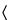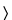Chlorophyllin Copper Complex Sodium
(klor'' oh fil' in kop' er).
DEFINITION
Chlorophyllin Copper Complex Sodium contains sodium salts of copper-chelated chlorophyll derivatives. It contains no artificial coloring.
IDENTIFICATION
Sample solution:  10 µg/mL
Medium:  pH 7.5 phosphate buffer, prepared by mixing 0.15 M dibasic sodium phosphate and 0.15 M monobasic potassium phosphate (21:4)
Acceptance criteria:  The ratio of A405/A630 is 3.0–3.9.
OTHER COMPONENTS
•  Content of Total Copper
Stock solution 1:  1000 µg/mL of copper. Transfer 1.000 g of copper to a 1000-mL volumetric flask, dissolve in 20 mL of nitric acid, and dilute with 0.2 N nitric acid to volume.[Note—Store in a polyethylene bottle. ]
Stock solution 2:  10 µg/mL of copper. Transfer 5.0 mL of Stock solution 1 into a 500-mL volumetric flask, and dilute with water to volume.
Standard solutions:  Transfer 5.0, 10.0, 15.0, and 20.0 mL, respectively, of Stock solution 2 to separate 100-mL volumetric flasks, and dilute the contents of each flask with water to volume. These Standard solutions contain 0.5, 1.0, 1.5, and 2.0 µg/mL of copper, respectively.
Sample solution:  Transfer 100 mg of previously dried Chlorophyllin Copper Complex Sodium to a Kjeldahl flask. Add 2.0 mL of sulfuric acid, 1.0 mL of nitric acid, and 1.0 mL of hydrogen peroxide, and carefully heat under a fume hood until a light green color is obtained. [Note—If the solution has any hint of a brown tint, continue to add 0.5-mL portions of nitric acid until a green color is obtained. ] Cool, transfer the contents quantitatively to a 1000-mL volumetric flask with several portions of water, dilute the contents of the flask with water to volume, and mix. Transfer 10.0 mL of this solution to a 50-mL volumetric flask, and dilute with water to volume.
Instrumental conditions
Mode:  Atomic absorption spectrophotometry
Lamp:  Copper hollow-cathode
Flame:  Air–acetylene
Analytical wavelength:  Copper emission line of 324.8 nm
Blank:  Water
Analysis
Samples:  Standard solutions and Sample solution
Determine the absorbances of the Standard solutions and the Sample solution. Plot the absorbances of the Standard solutions versus the concentration, in µg/mL, of copper, and draw the straight line best fitting the four plotted points. From the graph so obtained, determine the concentration, C, in µg/mL, of copper in the Sample solution.
Calculate the percentage of copper in the portion of Chlorophyllin Copper Complex Sodium taken:
Result = (C/W) × (V/F) × 100
 C = = concentration of the Sample solution determined from the graph (µg/mL) W = = weight of Chlorophyllin Copper Complex Sodium taken to prepare the Sample solution (mg) V = = final volume of Sample solution, 5000 mL F = = conversion factor, 1000 µg/mg
Acceptance criteria:  NLT 4.25% on the dried basis
•  Content of Chelated Copper
Analysis:  Calculate the percentage of chelated copper in the portion of Chlorophyllin Copper Complex Sodium taken by subtracting the percentage of ionic copper found in the test for Limit of Ionic Copper from the percentage of total copper found in the test for Content of Total Copper.
Acceptance criteria:  NLT 4.0% on the dried basis
•  Content of Sodium
Standard stock solution:  100 µg/mL of sodium. Dissolve 254.2 mg of sodium chloride, previously dried at 105for 2 h, in 50 mL of water. Transfer to a 1000-mL volumetric flask, and dilute with water to volume.
Standard solutions:  Transfer to each of four 100-mL volumetric flasks 10 mL of a nonionic wetting agent solution (1 in 500). To each flask add, respectively, 2.5, 5.0, 10.0, and 15.0 mL of the Standard stock solution, and dilute with water to volume. These Standard solutions contain 2.5, 5.0, 10.0, and 15.0 µg/mL of sodium, respectively.
Blank:  Transfer 10 mL of a nonionic wetting agent solution (1 in 500) into a 100-mL volumetric flask, and dilute with water to volume.
Sample solution:  Transfer 100 mg of Chlorophyllin Copper Complex Sodium to a 1000-mL volumetric flask. Add 100 mL of a solution of nonionic wetting agent (1 in 500) and 400 mL of water, and shake by mechanical means for 5 min. Dilute with water to volume.
Analysis
Samples:  Standard solutions and Sample solution
Set the flame photometer for maximum transmission at a wavelength of 589 nm. Adjust the instrument to zero transmittance with the Blank. Adjust the instrument to 100% transmittance with the most concentrated of the Standard solutions. Read the percentage of transmittance of the other Standard solutions, and plot the percentage of transmittance versus the concentration, in µg/mL, of sodium. Read the percentage of transmittance of the Sample solution, and from the graph read the concentration, C, in µg/mL, of sodium in the Sample solution.
Calculate the percentage of sodium in the portion of Chlorophyllin Copper Complex Sodium taken:
Result = (C/W) × (V/F) × 100
 C = = concentration of the Sample solution determined from the graph (µg/mL) W = = weight of Chlorophyllin Copper Complex Sodium taken to prepare the Sample solution (mg) V = = volume of Sample solution, 1000 mL F = = conversion factor, 1000 µg/mg
Acceptance criteria:  5%–7% on the dried basis
IMPURITIES
•  Limit of Ionic Copper
Standard solutions:  Prepare as directed in the test for Content of Total Copper.
Sample solution:  Transfer 100 mg of Chlorophyllin Copper Complex Sodium to a 150-mL conical flask. Add 75 mL of water, and shake by mechanical means for 3 min. Adjust with 1 N hydrochloric acid to a pH of 3.0, transfer the suspension thus obtained to a 100-mL volumetric flask, and dilute with water to volume. Filter this suspension, discarding the first 10 mL of the filtrate. Use the clear filtrate for analysis.
Analysis
Samples:  Standard solutions and Sample solution
Proceed as directed in the test for Content of Total Copper.
Calculate the percentage of ionic copper in the portion of Chlorophyllin Copper Complex Sodium taken:
Result = (C/W) × (V/F) × 100
 C = = concentration of the Sample solution determined from the graph (µg/mL) W = = weight of Chlorophyllin Copper Complex Sodium taken to prepare the Sample solution (in mg on the dried basis) V = = volume of Sample solution, 100 mL F = = conversion factor, 1000 µg/mg
Acceptance criteria:  NMT 0.25% on the dried basis
SPECIFIC TESTS
It meets the requirements of the tests for absence of Escherichia coli and Salmonella species.
9.5–10.7, in a solution (1 in 100)
Dry a sample at 150for 2 h: it loses NMT 5% of its weight.
•  Test for Fluorescence
Sample solution:   10 mg/mL
Analysis:   Apply 10 µL of Sample solution on filter paper, allow to dry, and examine the area of application under long-wavelength UV light through a red optical filter.
Acceptance criteria:  No fluorescence is visible.
•  Packaging and Storage: Preserve in tight, light-resistant containers.
Auxiliary Information— Please check for your question in the FAQs before contacting USP.
Topic/Question Contact Expert Committee
Monograph Feiwen Mao, M.S.
Senior Scientific Liaison
1-301-816-8320
(SM32010) Monographs - Small Molecules 361Radhakrishna S Tirumalai, Ph.D.
Principal Scientific Liaison
1-301-816-8339
(GCM2010) General Chapters - Microbiology62Radhakrishna S Tirumalai, Ph.D.
Principal Scientific Liaison
1-301-816-8339
(GCM2010) General Chapters - Microbiology
USP35–NF30 Page 2628
Pharmacopeial Forum: Volume No. 32(3) Page 769